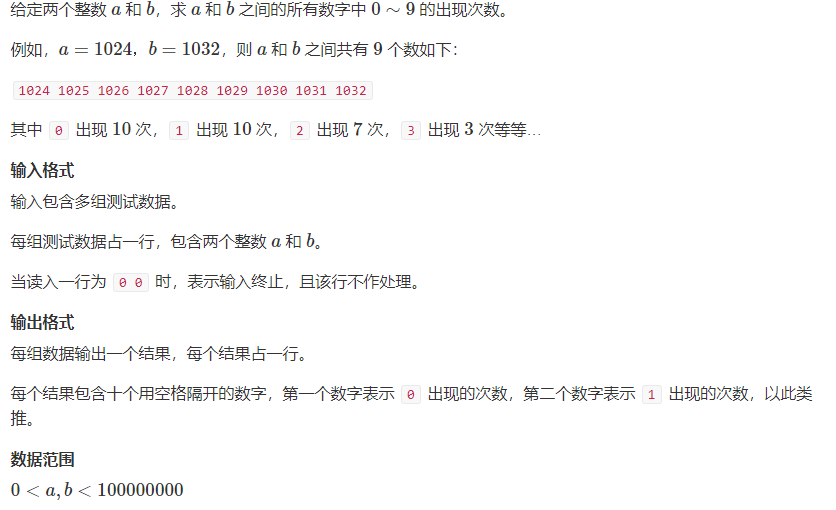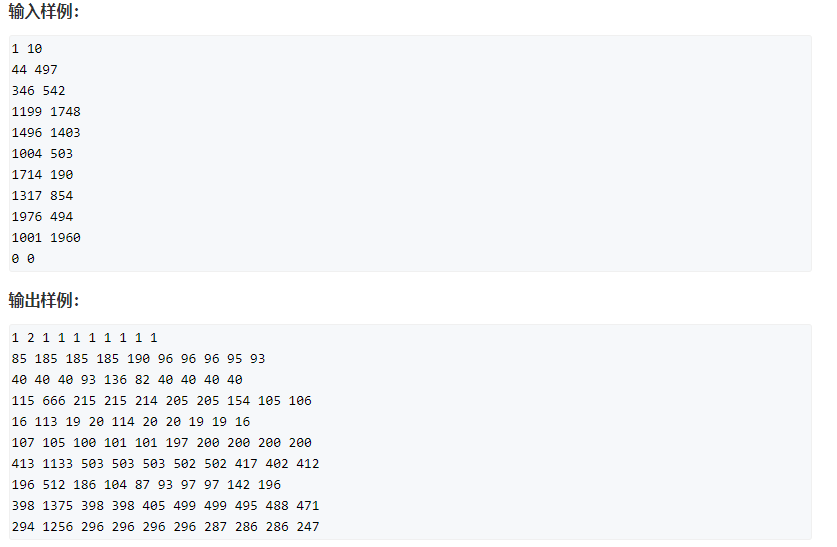# 数位统计DP

2021/7/20 14:32:17 浏览：

### 文章目录

• 前言
• 一、动态规划
• 二、AcWing 338. 计数问题
• 本题解析
• AC代码
• 三、时间复杂度

# 二、AcWing 338. 计数问题## 本题解析

`int count(int n, int x)`：返回在`1 ~ n``x`出现的次数
`int get(vector<int> num, int l, int r)`：返回`num`数组中从`r`位到`l`位的数是多少
`int power10(int x)`：返回`10``x`次方

(1) `xxx = 000 ~ abc - 1`：对于这种情况的话`yyy`的取值为：`000 ~ 999`，故这种的总情况数为：`abc * 1000`
(2) `xxx = abc`
(2.1) `d < 1`，那么`abc1yyy > abc0efg`，故这种没有符合情况的数
(2.2) `d = 1``yyy`的取值可为`000 ~ efg`，故这种的总情况数为：`efg + 1`
(2.3) `d > 1``yyy`的取值可为`000 ~ 999`，故这种的总情况数为：`1000`

`0`不会影响上述`count`函数实现中的 (2) 部分，只会对 (1) 部分产生影响：因为不可以出现形如`0123`这种数字

## AC代码

``````#include <iostream>
#include <algorithm>
#include <vector>

using namespace std;

const int N = 10;

int get(vector<int> num, int l, int r)
{
int res = 0;
for (int i = l; i >= r; i -- ) res = res * 10 + num[i];
return res;
}

int power10(int x)
{
int res = 1;
while (x -- ) res *= 10;
return res;
}

int count(int n, int x)
{
if (!n) return 0;

vector<int> num;
while (n)
{
num.push_back(n % 10);
n /= 10;
}
n = num.size();

int res = 0;
for (int i = n - 1 - !x; i >= 0; i -- )
{
if (i < n - 1)
{
res += get(num, n - 1, i + 1) * power10(i);
if (!x) res -= power10(i);       //对 0 的特判，即减去 001 的那一种情况
}

if (num[i] == x) res += get(num, i - 1, 0) + 1;
else if (num[i] > x) res += power10(i);
}

return res;
}

int main()
{
int a, b;
while (cin >> a >> b , a)
{
if (a > b) swap(a, b);    //题目中数据比较狗，可能出现 a > b 的情况，故需要特判一下

for (int i = 0; i <= 9; i ++ )
cout << count(b, i) - count(a - 1, i) << ' ';
cout << endl;
}

return 0;
}
``````